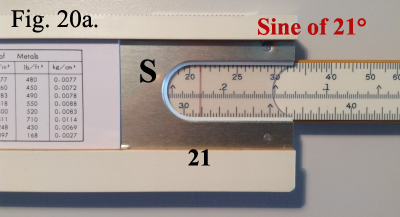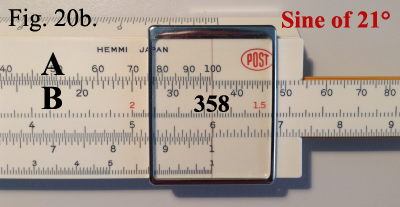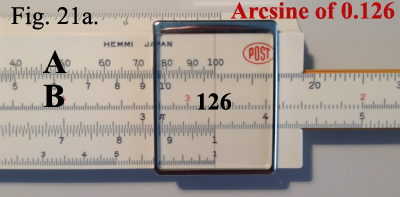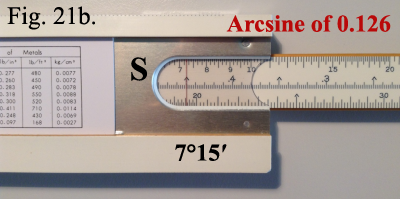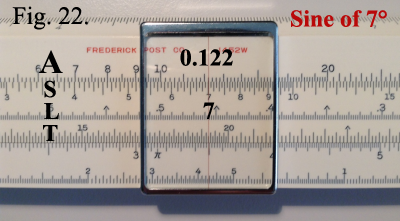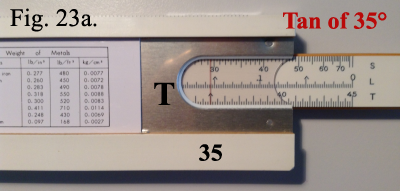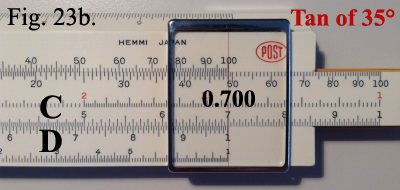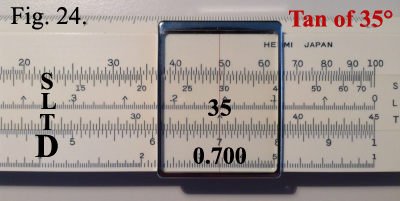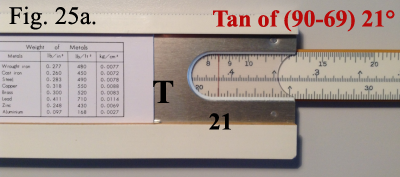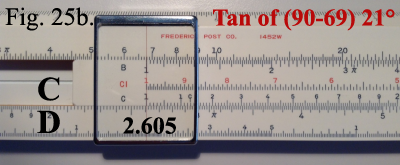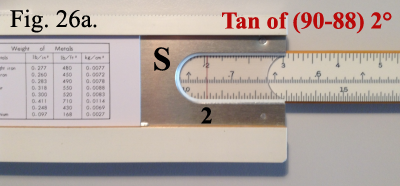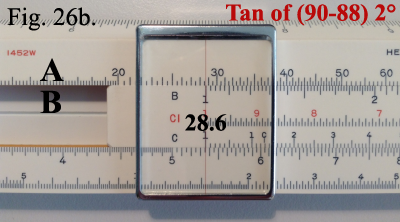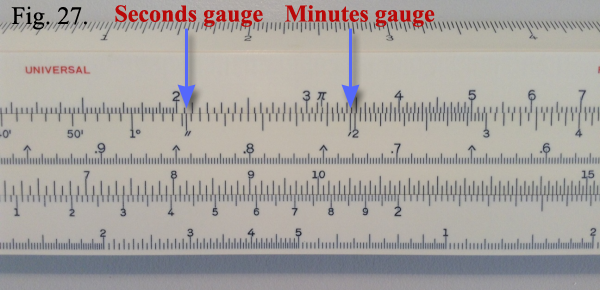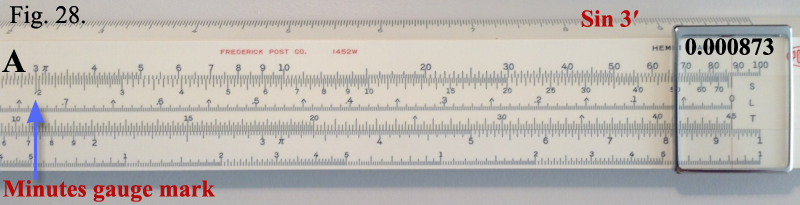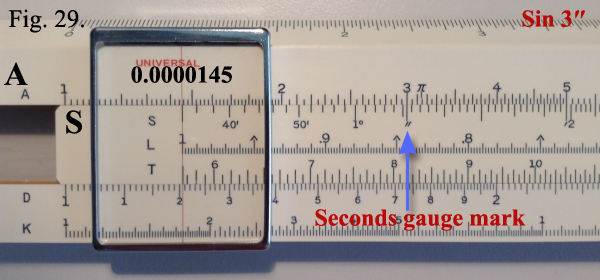TRIGONOMETRIC FUNCTIONS
 The two scales on the back of the slide marked S and T are the sine and tangent scales respectively. SinesThe S scale is divided to read in degrees and minutes from about 0°34′ to 90°. It is designed to be used with the A and B scales.Example: Find the sine of 21°.Set the slide to bring 21 on the S scale under the right-hand fixed hairline on the back of the rule. Example shown in Figure 20a. Turn the rule over, and read 358 on the B scale under the right index of the A scale. Example shown in Figure 20b.Note that the values of sines found on the right-hand half of the A and B scales range from 0.1 to 1.0 and those on the left-hand half range from 0.01 to 0.1. Therefore, our answer in this example is 0.358.When given the sine, the procedure is reversed to find the angle.Example: Find the angle whose sine is 0.126.Since the sine is greater than 0.1, 0.126 is located on the right-hand segment of the B scale. Draw the slide to bring 126 on the right half of the A scale. Example shown in Figure 21a. Turn the rule over, and read the angle 7°15′ on the S scale under the fixed hairline. Example shown in Figure 21b. Alternate Method (Sines)Remove the slide from the rule and re-insert so that the S, L en T scales are face up. With the slide centered so that the indices of the A scale line up with the ends of the S scale, sines can be read on the A scale directly opposite any angle on the S scale. Thus, to find the value of sin 7°, set the cursor hairline over 7 on the S scale and read the answer 0.122 under the hairline on the A scale. Example shown in Figure 22. TangentsThe T scale is divided in degrees and minutes from about 5°45′ to 45°. It is designed to be used with the C and D scales.Example: Find the value of tan 35°.Set 35 on the T scale under the right-hand fixed hairline on the back of the rule. Example shown in Figure 23a. Turn the rule over and read the answer 0.7000 on the C scale over the right index of the D scale. Example shown in Figure 23b. Note that the values of tangents of angles from 5°45′ to 45° range from 0.1 to 1.0. Alternate Method (Tangents)Turn the slide rule over as described in the alternate method for using the S scale and read directly from the T scale to the D scale or vice versa. Thus, to find the value of tan 35°, set the cursor hairline over 35 on the T scale and read the answer 0.007 under the hairline on the D scale. Example shown in Figure 24.

Tangents of Angles from 0°34′ to 5°45′The values of tangents and sines of angels smaller than 5°45′ are so nearly alike that they may be considered identical for slide rule computations. Consequently, for angles from 0°34′ to 5°45′, tangents can be read directly using the S scale and the left half of the A or B scales, as described in the section of sines.
Tangents of Angles greater than 45°Using the relationship
 tan x = 1 , tan (90 – x)
tangents for angles greater than 45° can be directly computed on the slide rule.Example: Find tan 69°.Solution: 90 – 69 = 21. Set 21 on the T scale under the right-hand fixed hairline. Example shown in Figure 25a. The answer, which is the reciprocal of tan 21, is read directly on the D scale under the left index of the C scale as 2.605. Example shown in Figure 25b. Note that for angles between 45° and approximately 84° the value of the tangent ranges from 1 to 10.When in the above relationship 90 – x is less than 5°45′, the left half of the S scale is used in making the computation. As pointed out above, the sines and tangents of small angles may be considered equal for slide rule purposes.Example: Find tan 88°.Set 2 on the S scale (90 – 88) under the right-hand fixed hairline. Example shown in Figure 26a. Turn the slide rule over and read the answer 28.6 on the A scale over the left index of the B scale. Example shown in Figure 26b. Using this method, tangents of angles up to 89°25′ can be read directly.
Sines and Tangents of Very Small AnglesFor determining the sine or tangent of a very small angle, two gauge points are provided on the S scale. The one identified by the symbol (′) is called the minutes gauge point and is fount next to the 2° mark on the S scale. The second gauge point marked (″) is found near the 1°10′ mark on the S scale. Example shown in Figure 27. They represent the value of the angle in radians. Their use is based on the fact that for very small angles, sin x = tan x = x (in radians), approximately.Example: Find sin 3′.Since sin 3′ = 3′ (in radians), solve 3 × 1′ (in radians). The procedure when using these gauge points is the same as the use of the CI and D scale combination for multiplication. With the slide rule turned so that the trig scales are face up, set the minutes gauge mark under the 3 on the A scale. Over the right index on the S scale, read 0.000873 on the A scale. Example shown in Figure 28.Example: Find sin 3″.Set the second gauge mark under 3 on the A scale. Over the left index on the S scale, read 0.0000145 on the A scale. Example shown in Figure 29.The decimal point in the above examples is located by noting that:1′ – 0.0003 radians (approximately)1″ – 0.000005 radians (approximately)